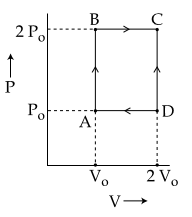## Filters

Sort by :
Clear All
Q
Ideal gas equation, PV = nRT n = w/M where w is weight of gas and M is molar mass PV = w/M (RT) => PM = w/V (RT)                    (density, d =w/V) => PM = dRT d = PM/RT d = 5x28/0.082x500 = 3.41 g/ml
Engineering
135 Views   |andare specific heats at constant pressure and constant volume respectively. It is observed thatfor hydrogen gasfor nitrogen gas

The correct relation between a and b is :

• Option 1)• Option 2)• Option 3)• Option 4)As we learnt in Specific heat capacity at constant pressure - - wherein f = degree of freedom R= Universal gas constant    Cp - Cv = a       (for hydrogen gas)  Cp - Cv = b        (for nitrogen gas)  Cp - Cv = R       ( Cp  & Cv   is molar specific heat) If we convert molar specific heat to specific heat a = 14 b Since mass of 1 mole of H2 = 2 g/mole  mass of 1 mole of N2 = 28 gm/mole Correct...
Engineering
114 Views   |

An external pressure P is applied on a cube at 00C so that it is equally compressed from all sides.  K is the bulk modulus of the material of the cube andis its coefficient of linear expansion.  Suppose we want to bring the cube to its original size by heating.  The temperature should be raised by :

• Option 1)• Option 2)• Option 3)• Option 4)As we learnt in Liquid - It is the type of matter which has got fixed volume but not fixed shape. Force of attraction between any two molecule is not that large as in case of solid .  - wherein e.g. Water, alcohol, milk etc.    Bulk modulus is defined as  Change in volume by heating  Since net change in volume is zero.      or        Correct option 1. Option 1) This is the correct...
Engineering
739 Views   |

An ideal gas has molecules with 5 degrees of freedom.  The ratio of specific heats at constant pressure (Cp) and at constant volume (Cv) is :

• Option 1)

6

• Option 2)• Option 3)• Option 4)As we learnt in Atomicity or adiabatic coefficient (gamma) -   - wherein for Monoatomic gas     for Diatomic gas          for Triatomic gas            f = 5  Correct option is 4. Option 1) 6 This is an incorrect option. Option 2) This is an incorrect option. Option 3) This is an incorrect option. Option 4) This is the correct option.
Engineering
875 Views   |

An engine operates by taking n moles of an ideal gas through the cycle ABCDA shown in figure.  The thermal efficiency of the engine is :

(Take Cv=1.5 R, where R is gas constant)• Option 1)

0.24

• Option 2)

0.15

• Option 3)

0.32

• Option 4)

0.08

As we learnt in Specific heat of gas at constant volume - - wherein f = degree of freedom R= Universal gas constant    Thermal Efficiency of Cycle  Gross Heat absorved is amount of heat that is absorbed only. These two are not the part of gross heat. For monoatomic gas f = 3 Correct option 2 Option 1)  0.24 This is an incorrect option. Option 2) 0.15 This is the correct option. Option...
Engineering
1607 Views   |

N moles of a diatomic gas in a cylinder are at a temperature T.  Heat is supplied to the cylinder such that the temperature remains constant but n moles of the diatomic gas get converted into monoatomic gas.  What is the change in the total kinetic energy of the gas ?

• Option 1)• Option 2)• Option 3)• Option 4)As we learnt in Specific heat of gas at constant volume - - wherein f = degree of freedom R= Universal gas constant    Number of moles of diatomic gas = N  Hence, Number of moles of monoatomic gas = 2N Since T = constant and volume is also constant  Work done = 0  Change in internal energy = Heat supplied Change in total kinetic energy = nfCvfT - niCviT ni = N,      nf = 2N,      Correct...
Exams
Articles
Questions